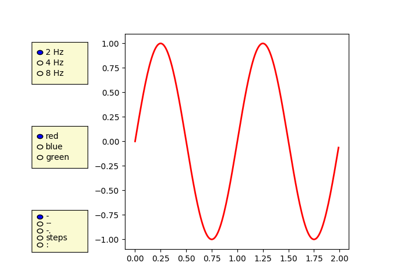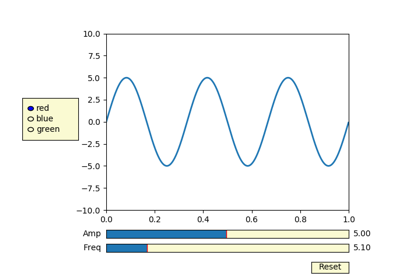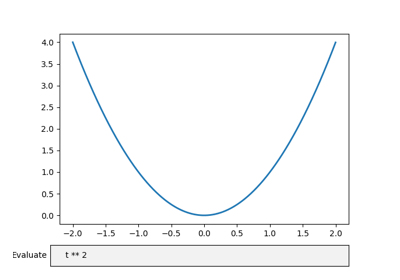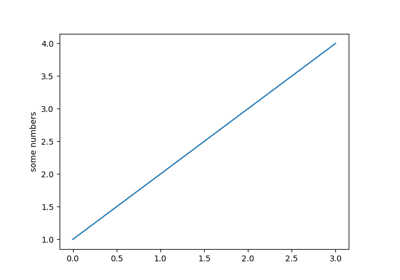### Related Topics

matplotlib.pyplot.subplots_adjust(left=None, bottom=None, right=None, top=None, wspace=None, hspace=None)[source]

Tune the subplot layout.

The parameter meanings (and suggested defaults) are:

left = 0.125  # the left side of the subplots of the figure
right = 0.9   # the right side of the subplots of the figure
bottom = 0.1  # the bottom of the subplots of the figure
top = 0.9     # the top of the subplots of the figure
wspace = 0.2  # the amount of width reserved for space between subplots,
# expressed as a fraction of the average axis width
hspace = 0.2  # the amount of height reserved for space between subplots,
# expressed as a fraction of the average axis height


The actual defaults are controlled by the rc file

## Examples using matplotlib.pyplot.subplots_adjust¶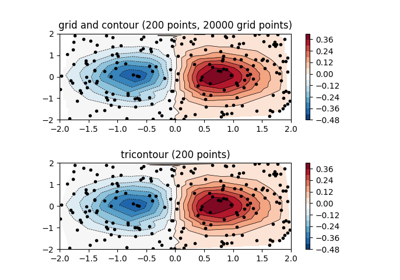Contour plot of irregularly spaced data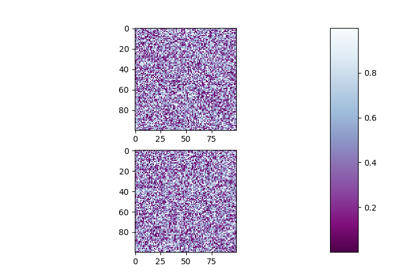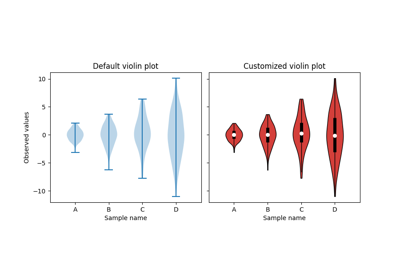Violin plot customization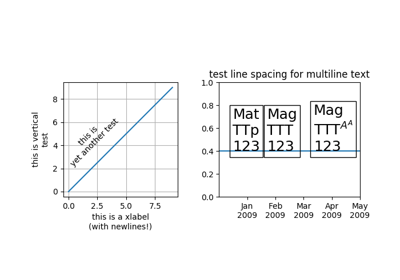Multiline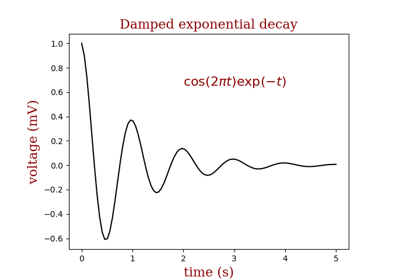Controlling style of text and labels using a dictionary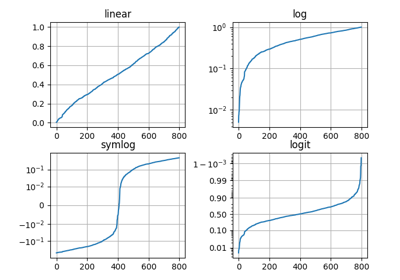Pyplot Scales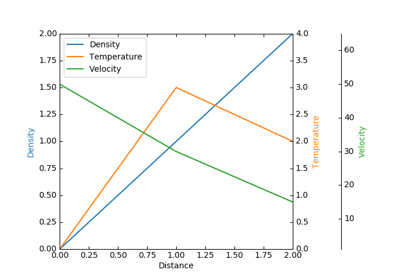Demo Parasite Axes2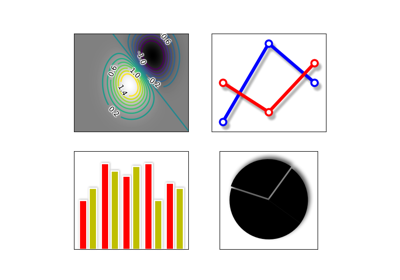Demo Agg Filter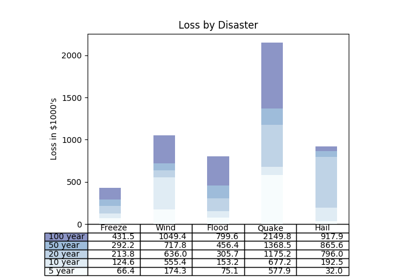Table Demo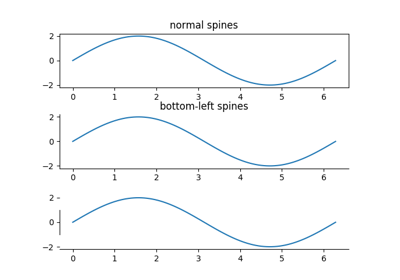Spines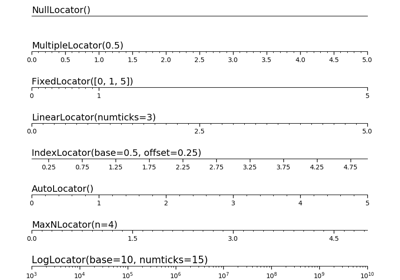Tick locators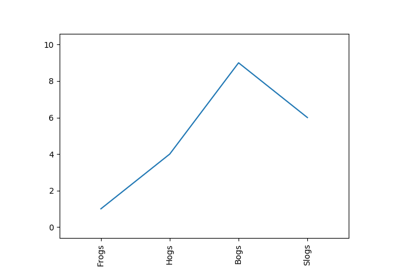Rotating custom tick labels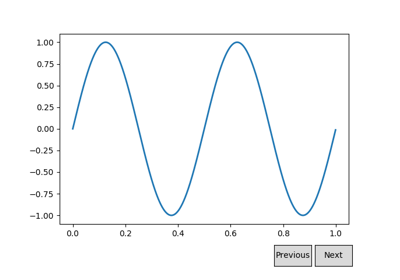Buttons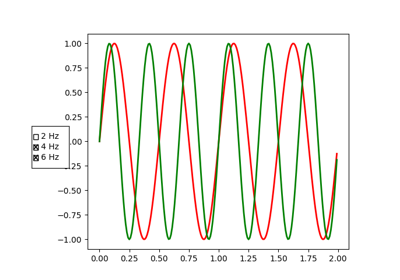Check Buttons